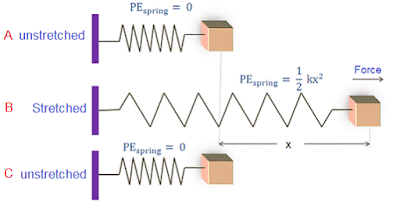## Pages

### 2.4 Potential Energy

In the previous section we discussed the Kinetic energy. Here we'll discuss the notion of Potential energy.

Basically, Potential energy is a capacity for doing work by virtue of the relative position. It is generally the property of the system and not of an individual body or particle. We will discuss about the three system that are of interest to us namely,
• Gravitational potential energy
• Electric potential energy
• Strain or elastic energy
Gravitational P.E: Gravitational P.E arises in systems of masses where the forces involved are gravitational attraction between them. Gravitational potential energy is generally referred to as potential energy.

The change in the potential energy $E_{p}$ of a mass $m$ that is moved through a vertical distance $h$ near the earth's surface where $g$ is the acceleration due to the gravitational attraction is defined as the work done on the mass to produce the move, and is given by
$$E_{p}=mgh$$
Electric P.E: "Electric potential energy arises in the system of charged particles where forces involved are repulsion between like charges and attractions between the opposite charges".

The change in the electric potential energy $E_{p}$ of a charge $q$ that is moved through a change in electric potential or potential difference $V$, is the work done on the charge to produce the move, and is mathematically given by,
$$E_{p}=qV$$
Elastic P.E: "It is the energy stored in the body by virtue of its elastic deformation."www.physics-and-radio-electronics.com

The elastic potential energy of a wire or spring of a natural length $l$ and displacement $x$ is equal to the work done in stretching the wire or spring from $l$ to $l+x$. Mathematically the Elastic potential energy for the spring is given by,
$$E_{p}=\frac{1}{2}kx^{2}$$
Here's an easy to understand video,
reference: cs toh Thomas cheong